# 1.9. Naive Bayes¶

Naive Bayes methods are a set of supervised learning algorithms based on applying Bayes’ theorem with the “naive” assumption of independence between every pair of features. Given a class variable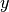and a dependent feature vector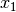through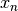, Bayes’ theorem states the following relationship: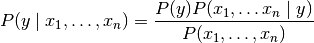Using the naive independence assumption that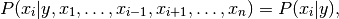for all, this relationship is simplified to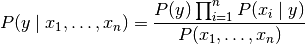Since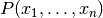is constant given the input, we can use the following classification rule: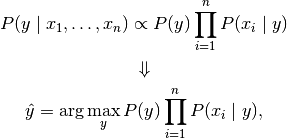and we can use Maximum A Posteriori (MAP) estimation to estimate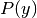and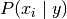; the former is then the relative frequency of classin the training set.

The different naive Bayes classifiers differ mainly by the assumptions they make regarding the distribution of.

In spite of their apparently over-simplified assumptions, naive Bayes classifiers have worked quite well in many real-world situations, famously document classification and spam filtering. They require a small amount of training data to estimate the necessary parameters. (For theoretical reasons why naive Bayes works well, and on which types of data it does, see the references below.)

Naive Bayes learners and classifiers can be extremely fast compared to more sophisticated methods. The decoupling of the class conditional feature distributions means that each distribution can be independently estimated as a one dimensional distribution. This in turn helps to alleviate problems stemming from the curse of dimensionality.

On the flip side, although naive Bayes is known as a decent classifier, it is known to be a bad estimator, so the probability outputs from predict_proba are not to be taken too seriously.

References:

## 1.9.1. Gaussian Naive Bayes¶

GaussianNB implements the Gaussian Naive Bayes algorithm for classification. The likelihood of the features is assumed to be Gaussian: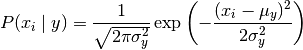The parameters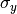and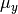are estimated using maximum likelihood.

>>> from sklearn import datasets
>>> from sklearn.naive_bayes import GaussianNB
>>> gnb = GaussianNB()
>>> y_pred = gnb.fit(iris.data, iris.target).predict(iris.data)
>>> print("Number of mislabeled points out of a total %d points : %d"
...       % (iris.data.shape,(iris.target != y_pred).sum()))
Number of mislabeled points out of a total 150 points : 6


## 1.9.2. Multinomial Naive Bayes¶

MultinomialNB implements the naive Bayes algorithm for multinomially distributed data, and is one of the two classic naive Bayes variants used in text classification (where the data are typically represented as word vector counts, although tf-idf vectors are also known to work well in practice). The distribution is parametrized by vectors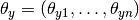for each class, where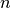is the number of features (in text classification, the size of the vocabulary) and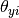is the probabilityof featureappearing in a sample belonging to class.

The parameters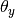is estimated by a smoothed version of maximum likelihood, i.e. relative frequency counting: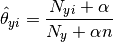where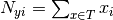is the number of times featureappears in a sample of classin the training set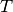, and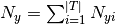is the total count of all features for class.

The smoothing priors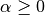accounts for features not present in the learning samples and prevents zero probabilities in further computations. Setting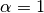is called Laplace smoothing, while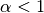is called Lidstone smoothing.

## 1.9.3. Bernoulli Naive Bayes¶

BernoulliNB implements the naive Bayes training and classification algorithms for data that is distributed according to multivariate Bernoulli distributions; i.e., there may be multiple features but each one is assumed to be a binary-valued (Bernoulli, boolean) variable. Therefore, this class requires samples to be represented as binary-valued feature vectors; if handed any other kind of data, a BernoulliNB instance may binarize its input (depending on the binarize parameter).

The decision rule for Bernoulli naive Bayes is based on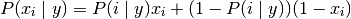which differs from multinomial NB’s rule in that it explicitly penalizes the non-occurrence of a featurethat is an indicator for class, where the multinomial variant would simply ignore a non-occurring feature.

In the case of text classification, word occurrence vectors (rather than word count vectors) may be used to train and use this classifier. BernoulliNB might perform better on some datasets, especially those with shorter documents. It is advisable to evaluate both models, if time permits.

References:

## 1.9.4. Out-of-core naive Bayes model fitting¶

Naive Bayes models can be used to tackle large scale classification problems for which the full training set might not fit in memory. To handle this case, MultinomialNB, BernoulliNB, and GaussianNB expose a partial_fit method that can be used incrementally as done with other classifiers as demonstrated in Out-of-core classification of text documents. Both discrete classifiers support sample weighting; GaussianNB does not.

Contrary to the fit method, the first call to partial_fit needs to be passed the list of all the expected class labels.

For an overview of available strategies in scikit-learn, see also the out-of-core learning documentation.

Note

The partial_fit method call of naive Bayes models introduces some computational overhead. It is recommended to use data chunk sizes that are as large as possible, that is as the available RAM allows.